Search Results
• #### 3. Given the unity feedback system, where G(s) = s(s +2) (s+3)(s +4) do the following:...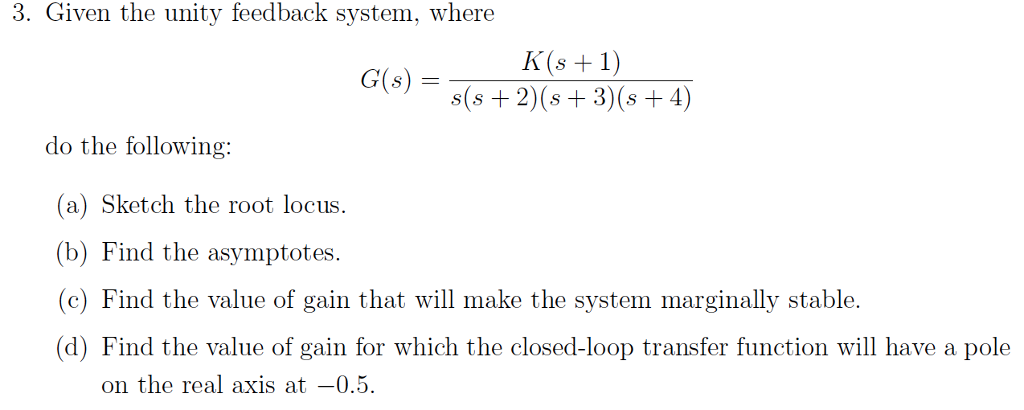3. Given the unity feedback system, where G(s) = s(s +2) (s+3)(s +4) do the following: (a) Sketch the root locus (b) Find the asymptotes c) Find the value of gain that will make the system marginally stable (d) Find the value of gain for which the closed-loop transfer function will have a pole on the real axis at-0.5

• #### s G1 = G2 = S-8 G2 s2+1 G3= G4 = R(s) C(s) S G1 G3...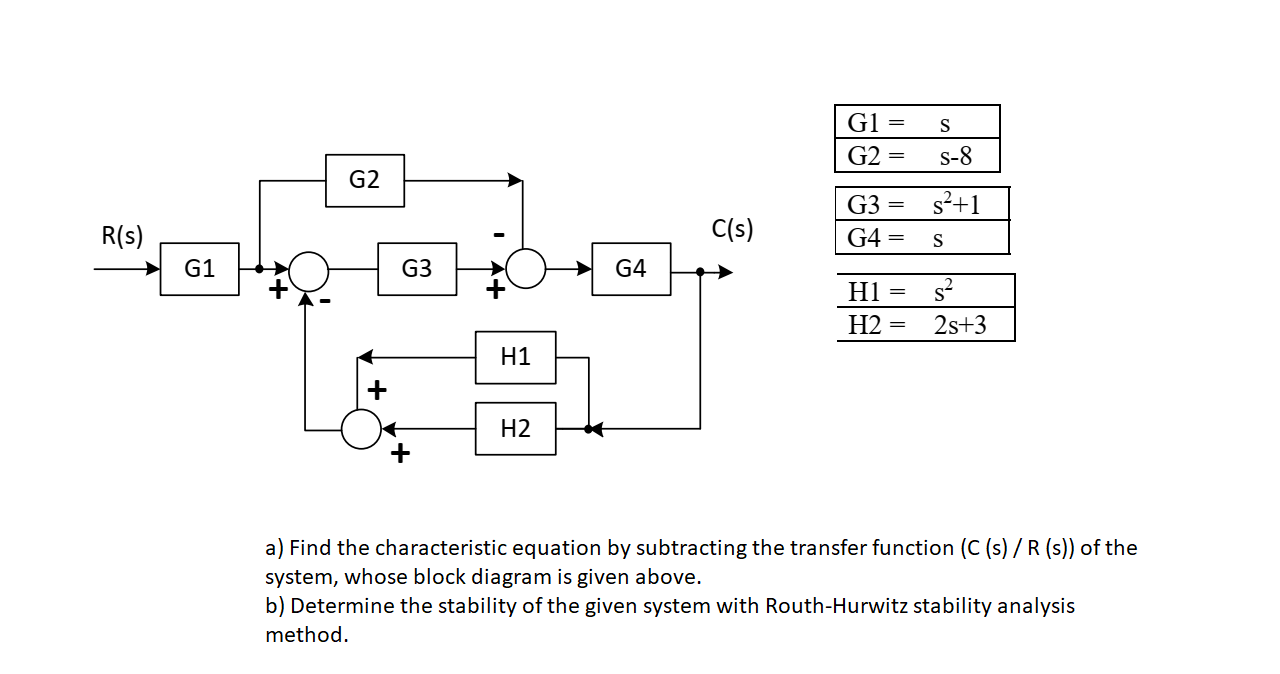s G1 = G2 = S-8 G2 s2+1 G3= G4 = R(s) C(s) S G1 G3 G4 H1 H2 si 28+3 H1 H2 a) Find the characteristic equation by subtracting the transfer function (C (s) / R (s)) of the system, whose block diagram is given above. b) Determine the stability of the given system with Routh-Hurwitz stability analysis method.

• #### Find the inverse Laplace transform of X(s) given that: x(s)= s(s+1) s? +58 +6 Note Degree...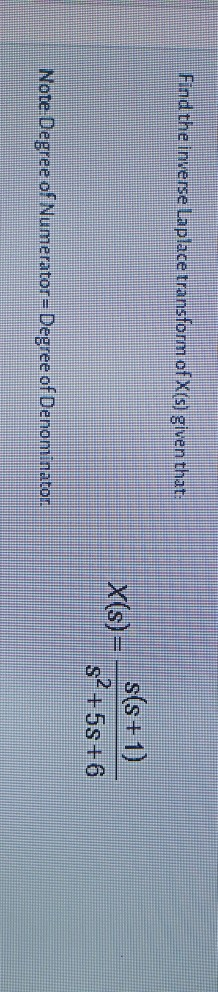Find the inverse Laplace transform of X(s) given that: x(s)= s(s+1) s? +58 +6 Note Degree of Numerator=Degree of Denominator.

• #### 1. Find t(s), n(s), b(s), k(s), T(s) for the following curves (don't forget to reparametrize by...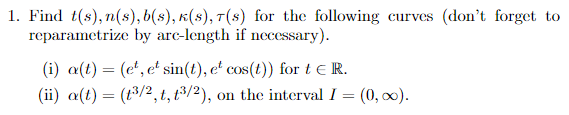1. Find t(s), n(s), b(s), k(s), T(s) for the following curves (don't forget to reparametrize by arc-length if necessary). (i) a(t) = (e', e' sin(t), e' cos(t)) for te R. (ii) a(t) = (13/2, t, t³/2), on the interval I = (0, o0).

• #### 7. In the Thermit reaction, 2Al(s) + Cr2O; (s) — 2Cr (s) + A1:0(s) a) What...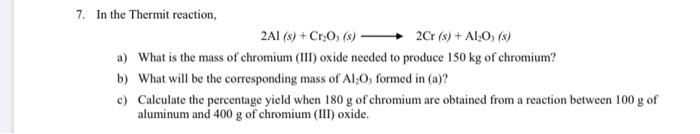7. In the Thermit reaction, 2Al(s) + Cr2O; (s) — 2Cr (s) + A1:0(s) a) What is the mass of chromium(III) oxide needed to produce 150 kg of chromium? b) What will be the corresponding mass of Al O, formed in (a)? c) Calculate the percentage yield when 180 g of chromium are obtained from a reaction between 100 g...

• #### 2. Find the inverse Laplace transform of (a) F(s) = e os s/(s – 2)(s? +...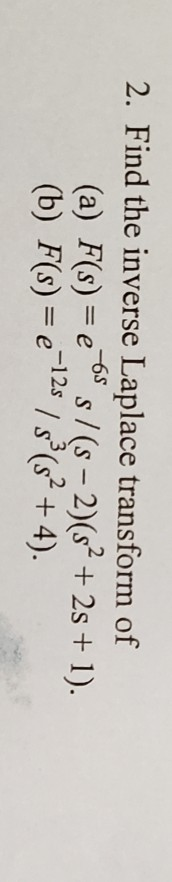2. Find the inverse Laplace transform of (a) F(s) = e os s/(s – 2)(s? + 25 + 1). (b) F(s) = e 128 / 8°(s? + 4).

• #### of S, namely, S- S = {m -n: m, n E S,m >n, is a set...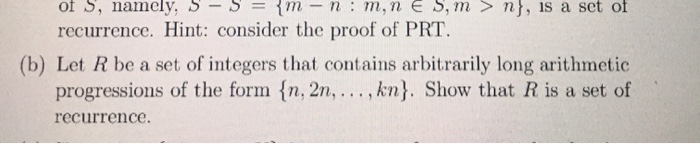of S, namely, S- S = {m -n: m, n E S,m >n, is a set of recurrence. Hint: consider the proof of PRT. (b) Let R be a set of integers that contains arbitrarily long arithmetic progressions of the form {n, 2n, ..., kn}. Show that R is a set of recurrence.

• #### Is the following function is a valued s- transform of pdf 1-e-s Mx(s) = S Select...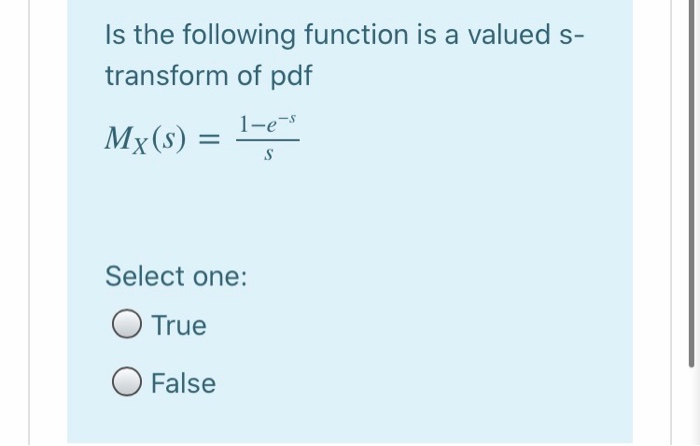Is the following function is a valued s- transform of pdf 1-e-s Mx(s) = S Select one: O True O False

• #### Transfer function of a motor is given by, K Es) s(s+a) o(s) where o(s) is the...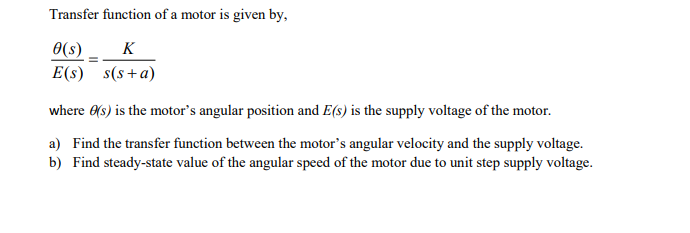Transfer function of a motor is given by, K Es) s(s+a) o(s) where o(s) is the motor’s angular position and E(s) is the supply voltage of the motor. a) Find the transfer function between the motor's angular velocity and the supply voltage. b) Find steady-state value of the angular speed of the motor due to unit step supply voltage.

• #### 2. [4pt] Sketch the Bode plots for the system below G(s) = S+ 2 s(s +...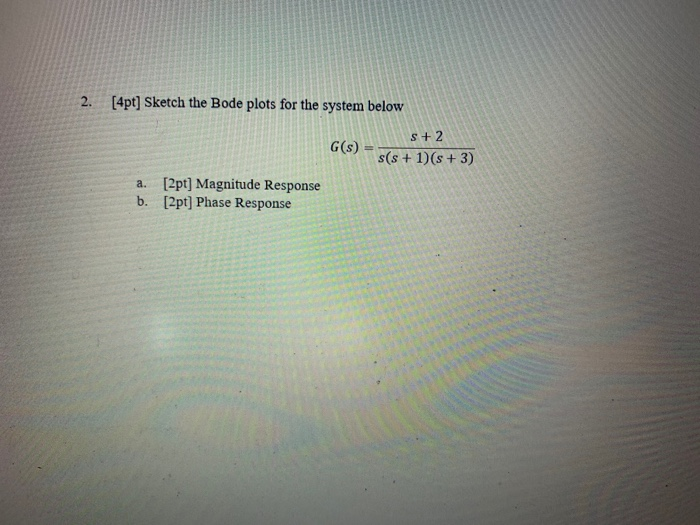2. [4pt] Sketch the Bode plots for the system below G(s) = S+ 2 s(s + 1)(s + 3) a. [2pt] Magnitude Response b. [2pt] Phase Response